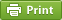Werner's Blog — Opinion, Analysis, Commentary
How regressive are fuel taxes in Canada?

Fuel and carbon taxes correct negative externalities. Such taxes are badly needed as market instruments to steer businesses and consumers towards more fuel efficiency and lower carbon intensity. A major concern with such taxes is their perceived regressivity: poorer households carry a disproportionately large share of the burden. Exactly how regressive are such taxes in Canada?

Measuring Regressivity and Progressivity

A measure of the progressivity or regressivity of a tax or expenditure is the Suits Index, which is closely related to another statistical measure—the Gini coefficient. Whereas the Gini coefficient captures income inequality by comparing the income of population quantiles, the Suits Index captures progressivity and regressivity by comparing the tax burden of income quantiles. Unlike the Gini coefficient, the Suits index can be in the range $$[-1,+1]$$ where negative numbers indicate regressivity and positive numbers indicate progressivity.

For continuous distributions, the Suits coefficient $$S$$ is calculated as the area difference between a 45-degree equal distribution line and the observed distribution. Then $S = 2\int_0^1 (y-T(y))\,\mathrm{d}y$ where $$y$$ is the cumulative share of income and $$T(y)$$ is the cumulative share of the tax burden. In practice we only have discrete observations of income and expenditures. We order the $$n$$ cumulative-income quantiles in ascending order so that $$y_{i-1} <y_i$$. Then the Suits index can be approximated over $$n$$ quantiles as $S=1-\sum_{i=1}^n [T(y_i)+T(y_{i-1})](y_i-y_{i-1})$ where $$y_{0}=0$$, $$y_n=1$$, $$T(0)=0$$ and $$T(1)=1$$. In practice, household expenditure surveys report data by household income quantiles where the population is divided into five or ten baskets (quintiles or deciles) in ascending order of income. Canada's Survey of Household Spending (SHS) reports results by quintiles. This generates an income share $$u_i$$ and an expenditure share $$v_i$$ for each quintile. Applying some math, one finds that $S = \sum_{i=1}^n u_i \left[ \sum_{k>i} v_k-\sum_{k<i}v_k \right]$ It is often practical to report the coefficient scaled up by 100, similar to the Gini coefficient.

Regressivity of Household Energy Expenditures

The table below shows the results of using income quintile data from Canada's SHS, averaged over the five-year 2012-2016 period in order to smooth out various data issues and fluctuations. Where "n/a" indicates non-availability , the product is either not available such as for natural gas in Quebec, or is not measured with sufficient confidence such as for gambling in B.C. and Alberta.

The benchmark for comparison is overall consumption across all goods. Consumption is regressive overall because savings, the difference between income and consumption, is strongly progressive. Canada's overall consumption has a regressivity of –11. Naturally, income taxes are strongly progressive (+27 for Canada), while property taxes are somewhat regressive (–6).

Groceries are typically among the most regressive (–17), although tobacco use is even more some (–30). Gambling expenditures are also highly regressive (–25).

Expenditure
Category
Suits Index (2012-16 Average)
Alcohol –4.4   –6.0   +0.4   –2.5   –13.9
Consumption –10.8   –12.6   –8.4   –10.4   –12.4
Electricity –18.3   –16.6   –14.5   –16.8   –19.6
Gambling –25.3   –25.5   –23.6   n/a   n/a
Groceries –17.1   –18.3   –12.5   –17.8   –18.4
Income Taxes +27.4   +28.8   +28.7   +26.4   +24.6
Motor Fuels –11.3   –11.8   –6.6   –9.3   –17.3
Natural Gas –8.0   –13.1   n/a   –10.0   –19.6
Property Taxes –6.3   –7.9   –1.6   –4.7   –10.8
Tobacco –29.6   n/a   –34.8   –17.5   –35.0
Transit –8.6   –13.2   –9.0   –9.1   –6.4

Energy in general is regressive, but motor fuels turn out to be less regressive than often thought. According to the statistics below, motor fuel purchases (mostly gasoline) are not all that different from overall consumption. This is a startling result that suggests that gasoline taxes are not particular regressive. By comparison, electricity purchases tend to be highly regressive overall in Canada (–17), although hydro-rich provinces Quebec and British Columbia have lower regressivity due to lower electricity costs. Natural gas tends to be regressive only where it is the primary heating source (Alberta).

Policy Implications

The surprising result that motor fuel expenditures are not overly regressive suggests that fuel taxes are not creating overly strong vertical equity problems. The poor are not paying disproportionately more than the rich when it comes to driving their motor vehicles. In part this may reflect that affluent people drive less fuel-efficient vehicles. Even if vertical equity is not such a big problem, there are significant concerns about horizontal equity. For example, in the Metro Vancouver region less affluent households tend to live further away from urban cares because of housing affordability. As a result, they tend to drive more to commute to work. Households with the same income level may experience different commuting patterns if households with children live in suburban areas and households without children live in urban cores closer to work opportunities. In order to address the implications of increasing fuel taxes, we need a better understanding (and better data about) horizontal equity.

Posted on Sunday, July 15, 2018 at 09:30 — #Economics | #EconometricsRecent Blog Entries

Topics

Months

Subscribe to RSS feed© 2023  Prof. Werner Antweiler, University of British Columbia.